# Software Projects

In addition to the theoretical exploration of new mathematical methods for solving linear systems, matrix equations and problems in ​​model order reduction or optimal control, we endeavor to create experimental as well as production implementations of our algorithms in software packages. The main focus is on the one hand to create reproducible results and on the other hand to make use of modern computer architectures and their programming models. Depending on the problem, we use one of the common programming languages ​​in scientific computing. These range from C / C++ and MATLAB to Fortran and Python in our research group and include complex projects that include several of those languages.

## Ongoing Developments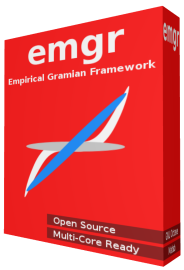#### emgr - EMpirical GRamian Framework

In system theory and control engineering the system Gramian matrices of linear input-output systems have wide-spread use, for example in: model reduction, decentralized control or sensitivity analysis. Empirical Gramians correspond to the linear system Gramians, but extend to parametric and nonlinear systems due to the purely data-driven computation. The empirical Gramian framework - emgr - is an open-source toolbox, compatible with OCTAVE and MATLAB, which enables the configurable computation of empirical system Gramians.
Beyond the computation of controllability, observability and minimality information on states, empirical Gramian matrices also allow the quantification of sensitivity and identifiability of parameters. Additionally, emgr provides low-rank computation, interfaces for integrators, inner products and kernels in a functional, vectorized and parallelizable implementation.
[more]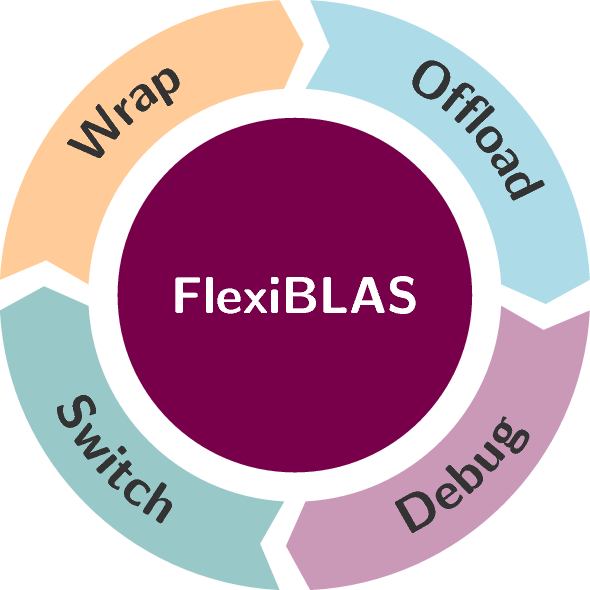#### FlexiBLAS - A Blas wrapper library with runtime exchangable backends

The BLAS library is one of the central libraries for the implementation of numerical algorithms. It serves as the basis for many other numerical libraries like LAPACK, PLASMA or MAGMA (to mention only the most obvious). Thus a fast BLAS implementation is the key ingredient for efficient  applications in this area. However, for debugging or benchmarking purposes it is often necessary to replace the underlying BLAS implementation of an application, e.g. to disable threading or to include  debugging symbols. We present a novel  framework that allows one to exchange the BLAS implementation at   run-time via an environment variable. Our concept neither requires relinkage, nor recompilation of the application. Numerical experiments show that there is no notable overhead introduced by this new approach. For only a very little overhead the framework naturally extends to a minimal profiling setup that allows one to count numbers of calls to the BLAS routines used and measure the time spent therein.
[more]#### GRANSO: GRadient-based Algorithm for Non-Smooth Optimization

GRANSO is an optimization package implemented in MATLAB, intended to be efficient for constrained nonsmooth optimization problems, without any special structure or assumptions imposed on the objective or constraint functions. It can handle problems involving functions that are any or all of the following: smooth or nonsmooth, convex or nonconvex, and locally Lipschitz or non-locally Lipschitz.
[more]#### HAPOD - Hierarchical Approximate Proper Orthogonal Decomposition

The hierarchical approximate proper orthogonal (HAPOD) decomposition enables the parallel (uniform, non-uniform, distributed) or memory-bound (embedded, single-board computer, compute-node) computation of PODs, for large or distributed data sets. The hapod function implements the distributed (star) and incremental (maximally unbalanced binary tree) topology variants of the HAPOD, and allows custom SVD (singular value decomposition) algorithms as backends. This compact POD tool is compatible with OCTAVE and MATLAB.
[more]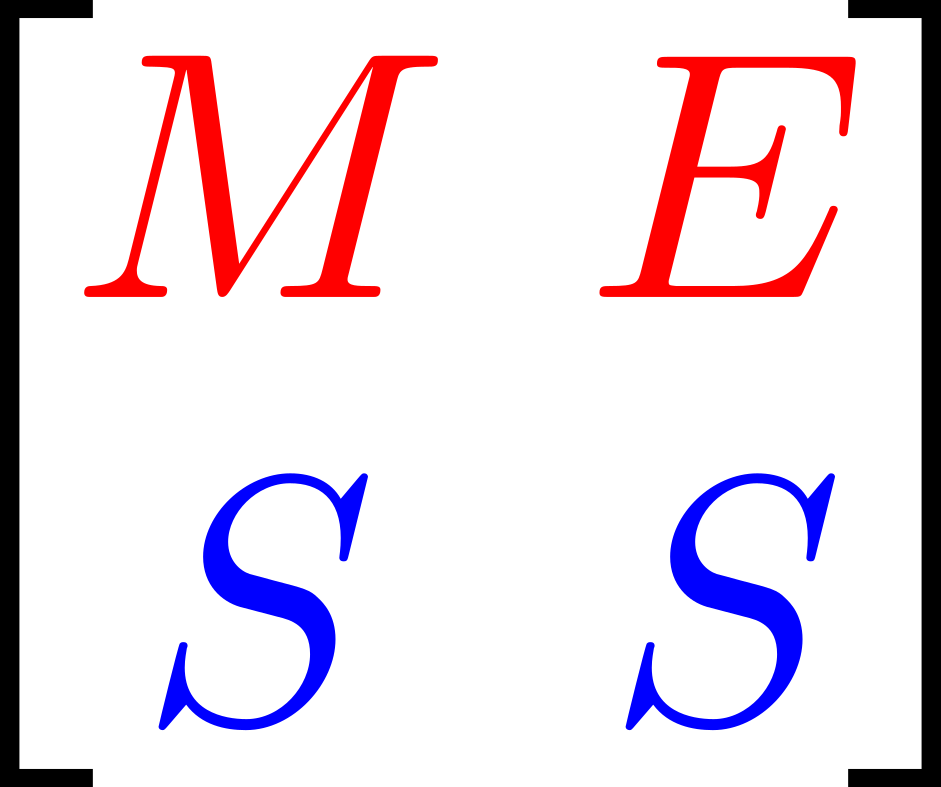#### Development of the parallel software packages (M.E.S.S.)

MESS, the Matrix Equations Sparse Solvers library, is the successor to the Lyapack Toolbox for MATLAB®. It will be available as a MATLAB toolbox as well as a C-library. It is intended for solving large sparse matrix equations as well as problems from model order reduction and optimal control. The C-version provides a large set of auxiliary subroutines for sparse matrix computations and efficient usage of modern multicore workstations.
[more]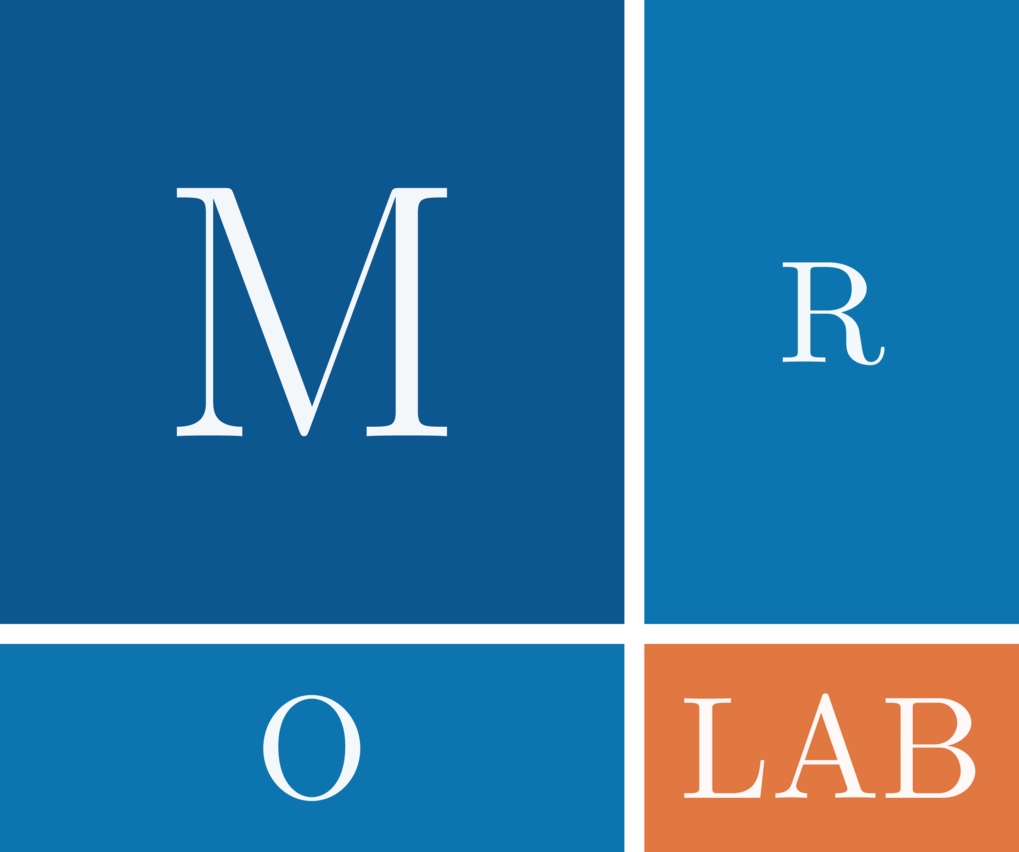#### MORLAB - Model Order Reduction LABoratory

The MORLAB toolbox is a collection of MATLAB/Octave routines for model order reduction of dynamical systems based on the solution of matrix equations. The implementation is made using spectral projection methods, e.g., methods based on the matrix sign function and the matrix disk function.
[more]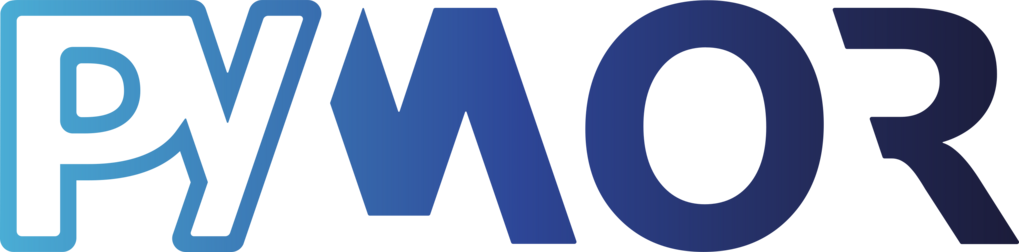#### pyMOR - Model Order Reduction with Python

pyMOR is a software library for building model order reduction applications with the Python programming language. Its main focus lies on the application of reduced basis methods to parameterized partial differential equations. All algorithms in pyMOR are formulated in terms of abstract interfaces for seamless integration with external high-dimensional PDE solvers. Moreover, pure Python implementations of finite element and finite volume discretizations using the NumPy/SciPy scientific computing stack are provided for getting started quickly.

The CSC group is extending the features to the class of dynamical systems with inputs and outputs.
[more]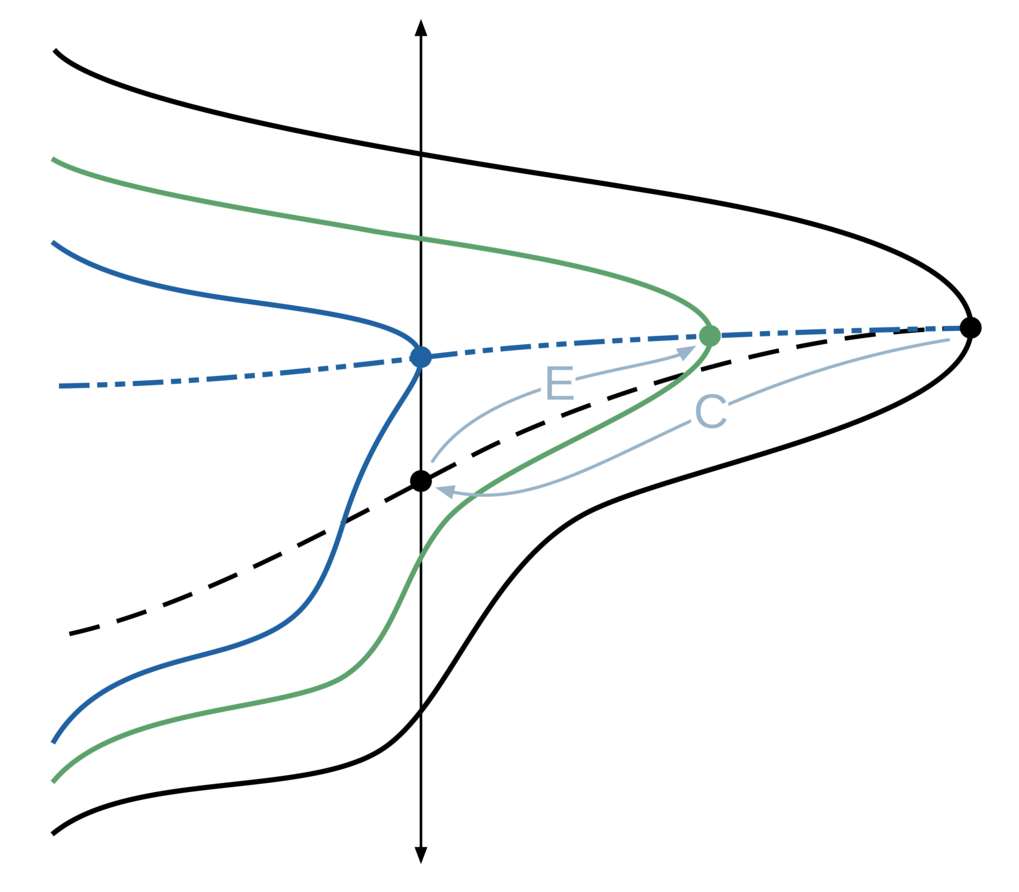#### ROSTAPACK: RObust STAbility PACKage

ROSTAPACK is a library implemented in MATLAB for computing or approximating robust stability measures, such as the H-infinity norm, of linear dynamical systems with input and output.
[more]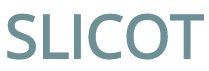#### The Control and Systems Library SLICOT

The subroutine library SLICOT provides Fortran 77 implementations of numerical algorithms for computations in systems and control theory. Based on numerical linear algebra routines from BLAS and LAPACK libraries, SLICOT provides methods for the design and analysis of control systems. Due to the use of Fortran 77, reusability of the software is obtained, so SLICOT can serve as the core for various existing and future CACSD platforms and production quality software. The further development is in cooperation with SynOptio GmbH.
[more]

## Concluded Developments

#### RRQR-MEX: A MATLAB MEX-interface for the rank revealing QR factorization

RRQR-MEX provides a MATLAB routine rrqr, implementing an interface to the FORTRAN RRQR codes by G. Quintana-Ortí and C.H. Bischof.
[more]

#### PLICMR - The Parallel Library in Control: Model Reduction

PLiCMR is a library of routines for parallel model reduction of large-scale discrete or continuous linear time invariant systems represented using the state-space model. Three methods are available in PLiCMR: Balance & Truncate, Singular Perturbation Approximation, and Hankel-Norm Approximation. Future extensions will include Balanced Stochastic Truncation.
[more]

#### Software for the solution of the Hamiltonian Eigenvalue Problem

The Hamiltonian Eigenvalue Problem occurs in many areas of Systems and Control theory. One of the main applications is the solution of the algebraic Riccati equation. We collect a set of FORTRAN 77 and MATALB Codes to solve this Eigenvalue problem and as well as the Riccati Equation.
[more]